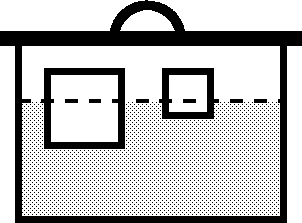Baltic Olympiad in Informatics
April 23-27, 2003, Tartu, Estonia

# Barrel

Some amount of water is poured into a barrel, then a number of cubes of different size and density are put into water. Finally, a lid is put onto the barrel and pushed down until it touches the edges of the barrel.Write a program to compute the resulting water level in the barrel.

It can be assumed that:

• the density of water is 1.0,
• the influence of air can be neglected,
• the cubes fit completely into the barrel,
• the cubes do not rotate and do not touch each other.

## Input data

The first line of the input file BARREL.IN contains three real numbers --- the bottom area of the barrel, S (0 < S ≤ 1000), the height of the barrel, H (0 < H ≤ 1000), and the volume of water, V (0 < V ≤ S∙H). The next line contains the number of cubes, N (0 < N ≤ 1000). It is followed by N lines, each containing two real numbers describing a cube --- the length of a side of the cube, L (0 < L ≤ 1000), and the density of the cube, D (0 < D ≤ 10).

## Output data

The first and only line of the output file BARREL.OUT must contain one real number --- the resulting water level. The output must not differ from the correct value by more than 10-4.

## Sample

 BARREL.IN BARREL.OUT 100 10 500 1 1 0.5 5.0050